# Below are production functions that turn capital (K) and labor (L) into output. For each of...

Below are production functions that turn capital (K) and labor (L) into output. For each of the production functions below, state and PROVE whether it is Constant/Increasing/or Decreasing Returns to scale. That is, you want to see how production changes when you increase all inputs (K,L,(M)) by a factor of α, where α > 1: (4 points each)

a) F(K,L) = K^1/3*L^1/3+2K+3

b) F(K,L) = sqr(K^3+L^3)

c) F(K,L) = (K^2/4+L^2/4)^2

d) F(K,L,M) = min(K,L)*M

Solution: a) This is not a homogeneous function. But analysing its each term we get that first term

K^1/3*L^1/3 follows decreaing returns to scale. Second term follows constant returns to scale. So overall F(K, L) = K^1/3*L^1/3+2K+3 follows Decreasing Returms to Scale.

b) F(K, L)= (K^3+L^3)^1/2

F(aK, aL)= a^3/2(K^3+L^3)^1/2= a^3/2F(K, L) > aF(K, L).

Hence it is an IRS production function.

c) F(K, L)= (K^2/4+L^2/4)^2

F(aK, aL)= a^2/4*2(K^2/4+L^2/4)^2= aF(K, L) = aF(K, L).

Hence it is an CRSproduction function.

d) F(K, L, M)= min(K, L)*M

F(aK, aL, aM)= a^2min(K,L)*M= a^2F(K, L) > aF(K, L).

Hence it is an IRS production function.

#### Earn Coin

Coins can be redeemed for fabulous gifts.

Similar Homework Help Questions
• ### 1. Below are production functions that turn capital (K) and labor (L) into output. For cach of th...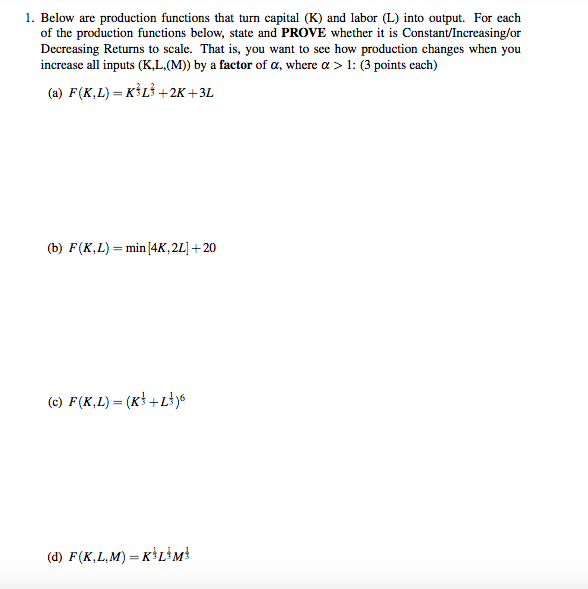1. Below are production functions that turn capital (K) and labor (L) into output. For cach of the production functions below, state and PROVE whether it is Constant/Increasing/or Decreasing Returns to scale. That is, you want to see how production changes when you increase all inputs (K,L, (M)) by a factor of a, where a > 1: (3 points each) (a) F(K,L)-KİLİ+2K +3L (b) F(K, L)=min/4K, 2L1+20 (d) F(K,L,M) KL3M 1. Below are production functions that turn capital (K) and...

• ### 1. Below are production functions that turn capital (K) and labor (L) into output. For each...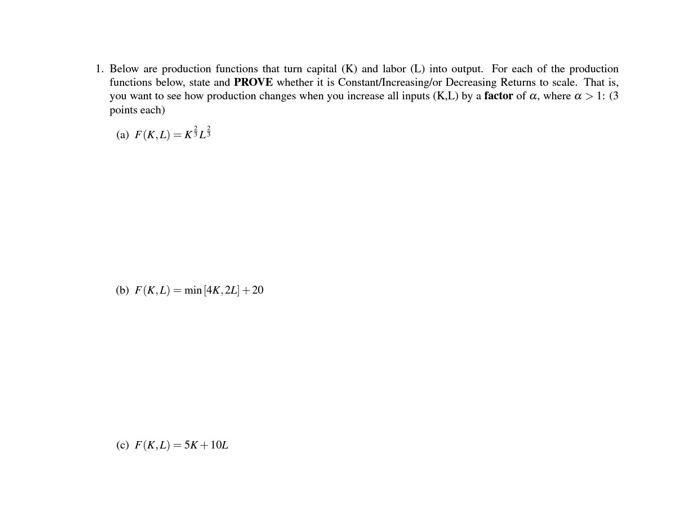1. Below are production functions that turn capital (K) and labor (L) into output. For each of the production functions below, state and PROVE whether it is Constant/Increasing/or Decreasing Returns to scale. That is, you want to see how production changes when you increase all inputs (K.L) by a factor of a, where a > 1: (3 points each) (a) F(KL)=KL (b) F(K,L) = min (4K, 22] + 20 (c) F(K,L) = 5K+10L

• ### 1. Below are production functions that turn capital (K) and labor (L) into output. For each...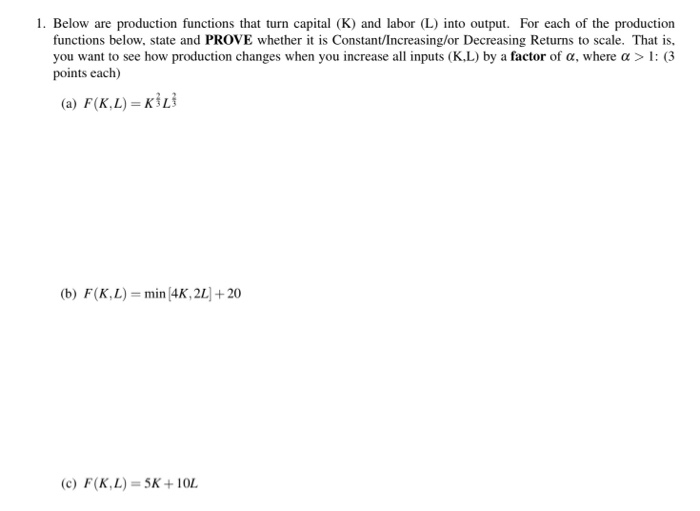1. Below are production functions that turn capital (K) and labor (L) into output. For each of the production functions below, state and PROVE whether it is Constant/Increasing/or Decreasing Returns to scale. That is, you want to see how production changes when you increase all inputs (KL) by a factor of a, where a > 1: (3 points each) (a) F(K.L) = (b) F(KL)= min (4K, 2L + 20 (c) F(K,L) = 5K+ 10L

• ### 4. Below are production functions that turn capital (K) and labor (L) into output. For each...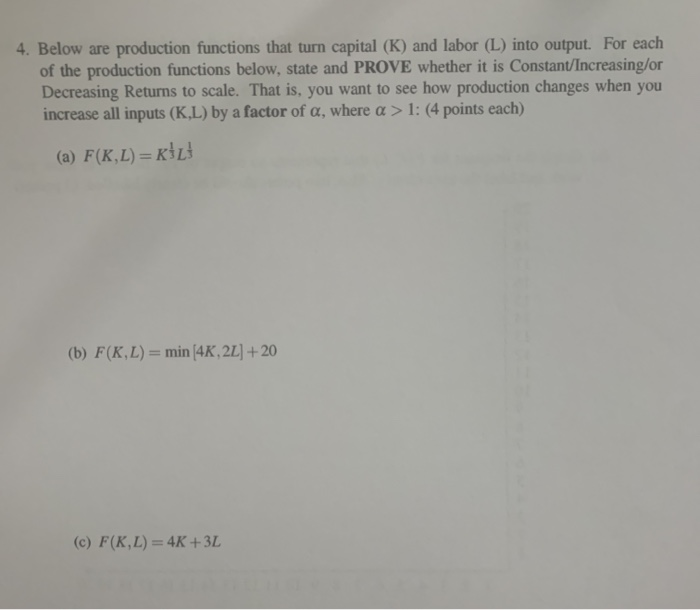4. Below are production functions that turn capital (K) and labor (L) into output. For each of the production functions below, state and PROVE whether it is Constant/Increasing/or Decreasing Returns to scale. That is, you want to see how production changes when you increase all inputs (K.L) by a factor of a, where a > 1: (4 points each) (a) F(K,L) =KİL (b) F(K,L) = min 4K, 2L] + 20 (c) F(K,L) = 4K +3L 5. For this problem you...

• ### 1. Graph the short-run total product curves for each of the following production functions if K...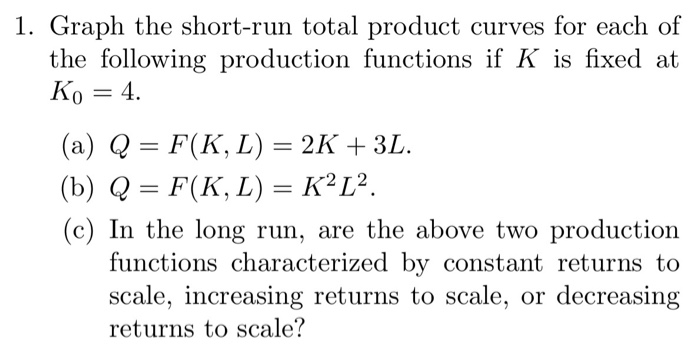1. Graph the short-run total product curves for each of the following production functions if K is fixed at Ko 4 (a) Q = F(K, L) = 2K + 3L. (b) Q = F(K, L) = K2L2. (c) In the long run, are the above two production functions characterized by constant returns to scale, increasing returns to scale, or decreasing returns to scale?

• ### 1. A production function is given by f(K, L) = L/2+ v K. Given this form,...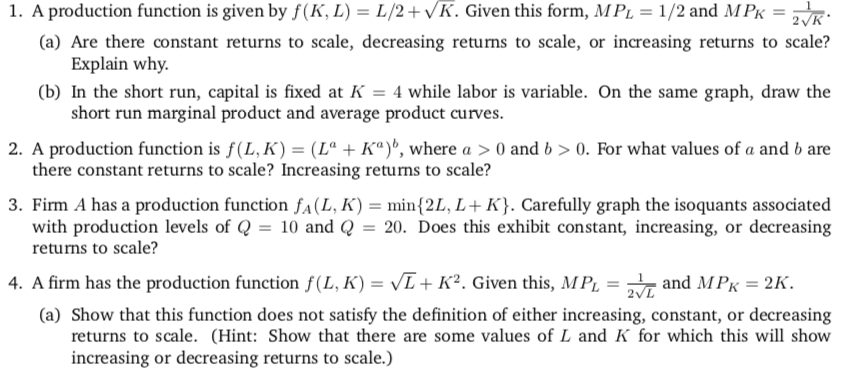1. A production function is given by f(K, L) = L/2+ v K. Given this form, MPL = 1/2 and MPK-2 K (a) Are there constant returns to scale, decreasing returns to scale, or increasing returns to scale? (b) In the short run, capital is fixed at -4 while labor is variable. On the same graph, draw the 2. A production function is f(LK)-(L" + Ka)", where a > 0 and b > 0, For what values of a and...

• ### 1. Returns to scale. A production function has constant returns to scale with respect to inputs w...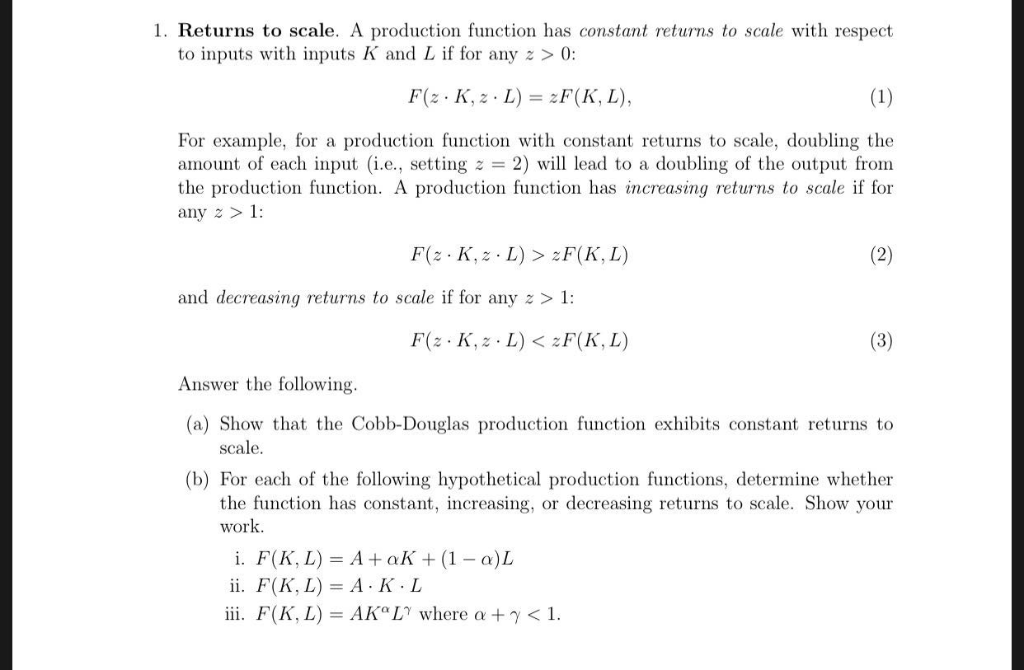1. Returns to scale. A production function has constant returns to scale with respect to inputs with inputs K and L if for any z >0: F(z . K, z、L) = zF(K, L), For example, for a production function with constant returns to scale, doubling the anount of each input (i.e., setting z = 2) will lead to a doubling of the output from the production function. A production function has increasing returns to scale if for any z and...

• ### 1. For the following production functions, find the marginal rate of technical substitu- tion (MRTSLK). Does the produc...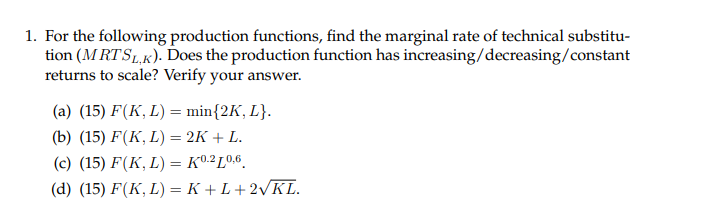1. For the following production functions, find the marginal rate of technical substitu- tion (MRTSLK). Does the production function has increasing/decreasing/constant returns to scale? Verify your answer (a) (15) F(K, L) = min{2K, L}. (b) (15) F(К, L) — 2K + L. (c) (15) F(K, L) = K0.2L0,6. (d) (15) F(K, L) — К +L+2VKL.

• ### Determine whether each of the production functions below displays constant, increasing, or decreasing returns to scale:...

Determine whether each of the production functions below displays constant, increasing, or decreasing returns to scale: Q = K + L + KL Q = 2K2 + 3L2 Q = KL Q = min(3K, 2L)

• ### Determine whether each of the production functions below displays constant, increasing, or decreasing returns to scale:...

Determine whether each of the production functions below displays constant, increasing, or decreasing returns to scale: Q = 10K0.75L0.25 Q = (K0.75L0.25)2 Q = K 0.75L0.75 Q = K 0.25L0.25 Q = K + L + KL Q = 2K2 + 3L2 Q = KL Q = min(3K, 2L)